# Electronics and Communication Engineering - Signals and Systems

Exercise : Signals and Systems - Section 6
1.
eAt can be expanded as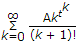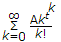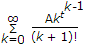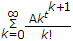Explanation:
No answer description is available. Let's discuss.

2.
If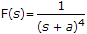, f(t) =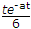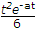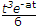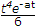Explanation:
No answer description is available. Let's discuss.

3.
For a wave v = V 1m sin (ωt + θ1 ) - V3m sin (3ωt + θ3), the rms value is (0.5 V21m + 0.5 V23m)0.5
True
False
Explanation:
No answer description is available. Let's discuss.

4.
The function in the given figure can be written as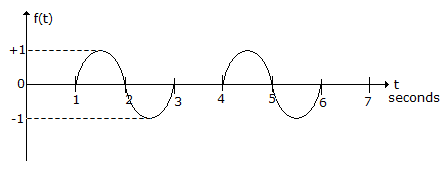u(t - 1) sin p ( t - 1) - u(t - 3) sin p (t - 3) + u(t - 4) sin p (t - 4) - u(t - 6) sin p (t - 6)
u(t - 1) sin p ( t - 1) - u(t - 3) sin p (t - 3) + u(t - 4) sin p (t - 4)
u(t - 1) sin p ( t - 1) + u(t - 4) sin p (t - 4)
u(t - 1) sin 2p ( t - 1) + u(t - 4) sin 2p (t - 4)